## 1. Linear Regression with Theano¶

The aim of this IPython notebook is to show some features of the Python Theano library in the field of machine learning. It has been developped by the LISA group at the University of Montreal (see: http://deeplearning.net/software/theano/). The notebook also relies on other standard Python libraries such as numpy, pandas and matplotlib.

To exemplify the use of Theano, this notebook solves the assignments of the Machine Learning MOOC provided by Coursera (see: https://www.coursera.org/learn/machine-learning) and performed in Stanford University by Andrew Ng (see: http://www.andrewng.org/).

The original MOOC assignments should to be programmed with the Octave language (see: https://www.gnu.org/software/octave/).The idea with this notebook is to provide Python developpers with interesting examples programmed using Theano.

This notebook has been developped using the Anaconda Python 3.4 distribution provided by Continuum Analytics (see: https://www.continuum.io/). It requires the Jupyter Notebook (see: http://jupyter.org/).

## 1.1 Linear regression with one variable¶

#### 1.1.1 Plotting the Data¶

In :
import pandas as pnd
import matplotlib.pyplot as plt
%matplotlib inline

df = pnd.read_csv('data/ex1data1.txt', header=None)
df.columns = ['X', 'Y']
df.plot(kind='scatter', x='X', y='Y', marker='x', s=40, color='red', figsize=(8,6))
plt.xlabel('Population of City in 10,000s', fontsize=9)
plt.ylabel('Profit in $10,000s', fontsize=9) plt.axis((4, 24, -5, 25)) plt.xticks(range(4,25,2));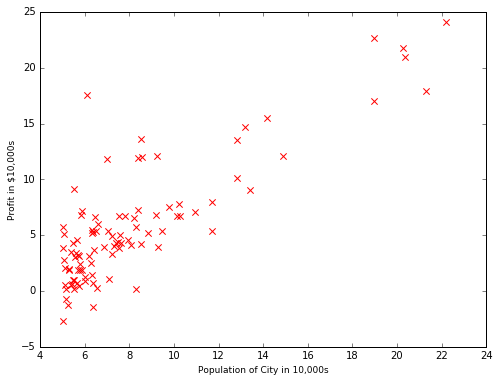#### 1.1.2 Gradient Descent¶ In : import theano import numpy as np import theano.tensor as T import matplotlib.pyplot as plt #Training Data m = df.shape X = np.matrix([np.ones(m), df["X"].values]) # Add a column of ones to x Y = df["Y"].values t = np.array([0.0, 0.0]) # initialize fitting parameters theta = theano.shared(t,name='theta') x = T.matrix('x') y = T.vector('y') prediction = T.dot(theta,x) cost = T.sum(T.pow(prediction-y,2))/(2*m) grad = T.grad(cost,theta) # Some gradient descent settings iterations = 1500 alpha = 0.01 train = theano.function([x,y],cost,updates = [(theta,theta-alpha*grad)]) test = theano.function([x],prediction) for i in range(iterations): costM = train(X,Y) if i==0: print("Cost(%i):" % i) print(costM) print("\nCost(%i):" % i) print(costM) print("\nTheta found by gradient descent:") print(theta.get_value()) # Plot the linear fit a = np.linspace(5,22,2) b = test(np.matrix([np.ones(a.shape), a])) plt.figure(figsize=(8,6)) plt.plot(a,b, label='Linear regression') plt.scatter(df["X"].values,Y, s=40,marker='x', color='r', label='Training data') plt.xlabel('Population of City in 10,000s', fontsize=9) plt.ylabel('Profit in$10,000s', fontsize=9)
plt.legend(loc=4, fontsize=9)
plt.axis((4, 24, -5, 25))
plt.xticks(range(4,25,2));

Cost(0):
32.072733877455654

Cost(1499):
4.483411453374869

Theta found by gradient descent:
[-3.63029144  1.16636235]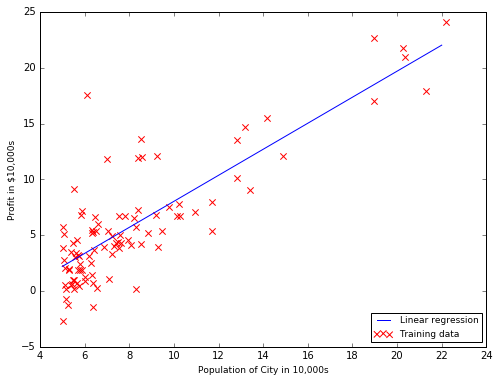In :
# Predict values for population sizes of 35,000 and 70,000
predict1 = np.dot(np.array([1, 3.5]), theta.get_value())
print('For population = 35,000, we predict a profit of %f' % (predict1*10000))
predict2 = np.dot(np.array([1, 7]), theta.get_value())
print('For population = 70,000, we predict a profit of %f' % (predict2*10000))

For population = 35,000, we predict a profit of 4519.767868
For population = 70,000, we predict a profit of 45342.450129


#### 1.1.3 Visualizing J($\theta$)¶

In :
# Grid over which we will calculate J
theta0_vals = np.linspace(-10, 10, 100)
theta1_vals = np.linspace(-1, 4, 100)

# initialize J_vals to a matrix of 0's
J_vals = np.zeros((theta0_vals.shape, theta1_vals.shape))

# Fill out J_vals
for i, theta0 in enumerate(theta0_vals):
for j, theta1 in enumerate(theta1_vals):
theta_ = np.array([theta0, theta1])
J_vals[j,i] = np.sum(np.power(np.dot(theta_,X)-Y,2))/(2*m)

# Surface cost function
from mpl_toolkits.mplot3d import Axes3D
from matplotlib import cm
x_vals, y_vals = np.meshgrid(theta0_vals, theta1_vals)
fig = plt.figure(figsize=(8,6))
ax = fig.gca(projection='3d')
surf = ax.plot_surface(y_vals, x_vals, J_vals, rstride=1, cstride=1, linewidth=0, cmap=cm.jet);
# invert x axis to get the same 3D representation as in Octave
ax.invert_xaxis()
plt.xlabel(r'$\theta_{1}$')
plt.ylabel(r'$\theta_{0}$');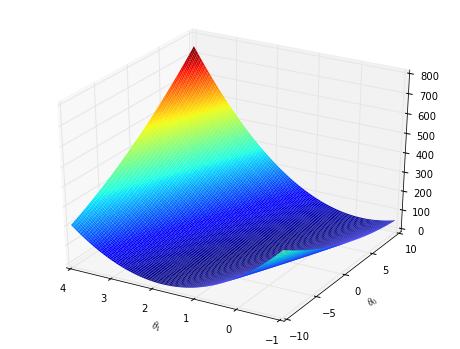In :
# Contour cost function, showing minimum
levels = [i*i for i in np.arange(25)]
contour = plt.contour(theta0_vals, theta1_vals, J_vals, levels)
plt.plot(theta.get_value(), theta.get_value(), 'rx')
plt.xlabel(r'$\theta_{0}$')
plt.ylabel(r'$\theta_{1}$')
plt.xticks(np.arange(-10,11,2))
plt.yticks(np.arange(-1,4.5,0.5));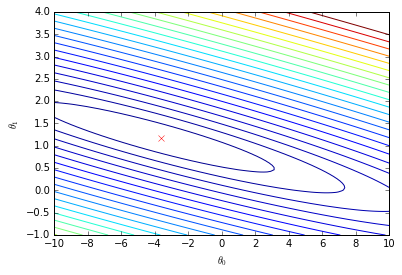### 1.2 Linear regression with multiple variables¶

In :
df2 = pnd.read_csv('data/ex1data2.txt', header=None)
df2.columns = ['X1', 'X2', 'Y']
print('First 10 examples from the dataset:')
for i in range(10):
print(' x = [%i, %i], y = %i ' % (df2.loc[i, 'X1'], df2.loc[i, 'X2'], df2.loc[i, 'Y']))

First 10 examples from the dataset:
x = [2104, 3], y = 399900
x = [1600, 3], y = 329900
x = [2400, 3], y = 369000
x = [1416, 2], y = 232000
x = [3000, 4], y = 539900
x = [1985, 4], y = 299900
x = [1534, 3], y = 314900
x = [1427, 3], y = 198999
x = [1380, 3], y = 212000
x = [1494, 3], y = 242500


#### 1.2.1 Feature Normalization¶

In :
mu = df2.mean()
sigma = df2.std()
df3 = (df2 - mu)/sigma


#### 1.2.2 Gradient Descent¶

In :
#Training Data
m = df3.shape
Xnorm = np.matrix([np.ones(m), df3["X1"].values, df3["X2"].values]) # Add intercept term to X
Y = df2["Y"].values

# Choose some alpha value
alpha = 0.01
num_iters = 5000

# Init Theta and Run Gradient Descent
t = np.zeros(3)
theta = theano.shared(t,name='theta')

x = T.matrix('x')
y = T.vector('y')

prediction = T.dot(theta,x)
cost = T.sum(T.pow(prediction-y,2))/(2*m)
grad = T.grad(cost,theta)
train = theano.function([x,y],cost,updates = [(theta,theta-alpha*grad)])

for i in range(num_iters):
costM = train(Xnorm,Y)

print("Theta found by gradient descent:")
print(theta.get_value())

Theta found by gradient descent:
[ 340412.65957447  110631.05025395   -6649.47424592]

In :
# Predict values for 1650 sq-ft and 3 bedrooms
# Need to normalize data
X0 = np.array([1, (1650 - mu['X1'])/sigma['X1'], (3 - mu['X2'])/sigma['X2']])
price = np.dot(theta.get_value(), X0)
print('Predicted price of a 1650 sq-ft, 3 br house (using gradient descent):\n$%f' % price)  Predicted price of a 1650 sq-ft, 3 br house (using gradient descent):$293081.464340


#### 1.2.3 Selecting learning rates¶

In :
num_iters = 50

x = T.matrix('x')
y = T.vector('y')

alphas = [0.01, 0.03, 0.10, 0.30]
colors = ['k', 'r', 'g', 'b']
l = len(alphas)
costM = np.zeros((l, 50))
for j, alpha in enumerate(alphas):
# Init Theta and Run Gradient Descent
t = np.zeros(3)
theta = theano.shared(t,name='theta')
prediction = T.dot(theta,x)
cost = T.sum(T.pow(prediction-y,2))/(2*m)
grad = T.grad(cost,theta)
train = theano.function([x,y],cost,updates = [(theta,theta-alpha*grad)])
for i in range(num_iters):
costM[j, i] = train(Xnorm,Y)

plt.figure(figsize=(8,6))

for j, alpha in enumerate(alphas):
plt.plot(costM[j], color=colors[j], label = r'$\alpha = %.2f$' % alpha)

plt.xlabel('Number of iterations', fontsize=9)
plt.ylabel('Cost J', fontsize=9)
plt.legend(fontsize=10)
plt.xticks(range(0,51,5));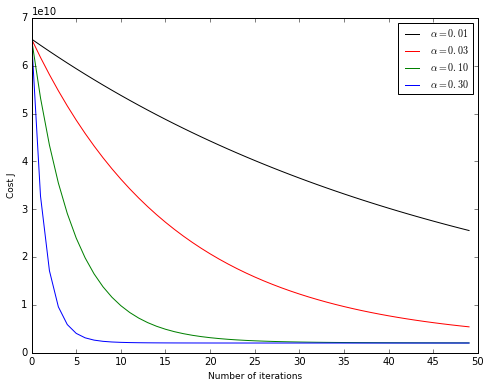#### 1.2.4 Normal Equations¶

In :
from numpy.linalg import pinv
# Add intercept term to X
X = np.matrix([np.ones(m), df2["X1"].values, df2["X2"].values])
# Calculate the parameters from the normal equation
theta = np.dot(np.dot(pinv(np.dot(X.T, X)),X.T).T, Y)
print('Theta computed from the normal equations:')
print(theta)
X0 = np.array([1, 1650, 3])
price = np.dot(theta, X0)
print('\nPredicted price of a 1650 sq-ft, 3 br house (using normal equations):\n$%f' % price)  Theta computed from the normal equations: [[ 89597.90963335 139.21067408 -8738.01914598]] Predicted price of a 1650 sq-ft, 3 br house (using normal equations):$293081.464430